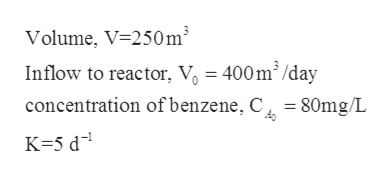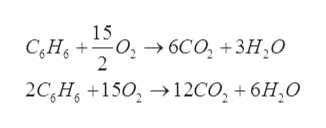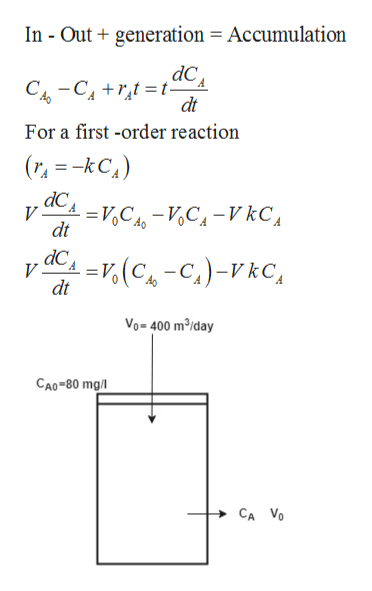# An industry is planning to use a 250 m3 bioreactor to treat 400 m3/day of wastewater containing 80 mg/L of benzene (CsHe). Benzene is expected to degrade by microbial action in a completely-mixed reactor (CSTR) with a first-order degradation rate coefficient of 5.0/ day a. Write a balanced reaction for the complete oxidation of benzene to carbon dioxide and water. b. Draw a diagram and write a mass balance equation for benzene in the tank reactor. c. Assuming steady-state conditions, determine the concentration of benzene in the outflow d Determine the mass of benzene that comes into the reactor each day, the mass of benzene that goes out of the reactor each day, and the mass of benzene that is degraded each day. e. Calculate the percent removal. If air is com bubbled oxygen transfer efficiency is equal to 15%. posed of 21 percent oxygen, estimate the volume of air that would have to be through the reactor each day. Assume the air temperature is 25°C and that the Ans: c) 19.4 mg/L d) mass rate in -32.0 kg/day, mass rate out 7.8 kg/day, mass rate loss by reactions 24.2 kg/day, e) 75.8% f) 1,809 m3 air/day

Question
10 views

An industry is planning to use a 250 m3 bioreactor to treat 400 m3/day of wastewater containing 80 mg/L of benzene (CsHe). Benzene is expected to degrade by microbial action in a completely-mixed reactor (CSTR) with a first-order degradation rate coefficient of 5.0/ day a. Write a balanced reaction for the complete oxidation of benzene to carbon dioxide and water. b. Draw a diagram and write a mass balance equation for benzene in the tank reactor. c. Assuming steady-state conditions, determine the concentration of benzene in the outflow d Determine the mass of benzene that comes into the reactor each day, the mass of benzene that goes out of the reactor each day, and the mass of benzene that is degraded each day. e. Calculate the percent removal. If air is com bubbled oxygen transfer efficiency is equal to 15%. posed of 21 percent oxygen, estimate the volume of air that would have to be through the reactor each day. Assume the air temperature is 25°C and that the Ans: c) 19.4 mg/L d) mass rate in -32.0 kg/day, mass rate out 7.8 kg/day, mass rate loss by reactions 24.2 kg/day, e) 75.8% f) 1,809 m3 air/day

check_circle

Step 1

As per honor code only three sub-parts are answered at a time so please upload separately for remaining parts.

Given information:help_outlineImage TranscriptioncloseVolume, V 250m3 Inflow to reactor, V, = 400m3/day concentration ofbenzene, C =80mg/L K-5 d fullscreen
Step 2

a).Balanced equation is writes ashelp_outlineImage Transcriptionclose15 -0, > 6со, +3Н,о 2 C,Н, + 2C, Н, +150, >12со, + 6H,0 fullscreen
Step 3

b).A mole balance equati...help_outlineImage TranscriptioncloseIn - Out generation Accumulation ас CCAtt- dt A For a first -order reaction (rkC) =VC C-VkC dt ас V =,(C-C)-kC dt Vo-400 m2/day CAO-80 mg/l CA Vo fullscreen

### Want to see the full answer?

See Solution

#### Want to see this answer and more?

Solutions are written by subject experts who are available 24/7. Questions are typically answered within 1 hour.*

See Solution
*Response times may vary by subject and question.
Tagged in
EngineeringCivil Engineering

### Environmental Science